# Excel 2010: Using Array Formula

Excel provides a way to deal with arrays. Sometimes it becomes a frantic need to apply formula, or do any other operation using arrays. By using arrays you can deal with two list of data simultaneously. Excel Array formulas empowers user to deal with series of data/values rather than handling single data value. In this post we will apply simple array operation over the data cells for finding out SUM of values.

Launch Excel 2010, and open a datasheet on which you want to apply Array operation. To start off with using array, we have included a datasheet, containing fields, Name, Item, and Price as shown in the screenshot below.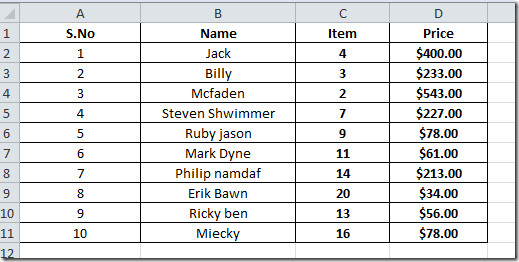Now we need to evaluate the sum of all the product price, for this we need to multiply each item sold with its respective price. For example, we need to multiply each item with its price and show the result (4*400=1600, 3*233 and so on).

For this, we need to perform array operation over it. First we will give cell a label Total Sale, beneath the table.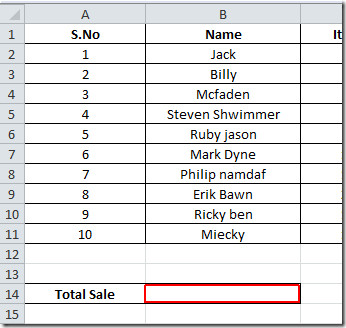Now we will write a formula in the cell, adjacent to Total Sale.

The syntax of simple SUM formula is;

=SUM(table_range*table_range)

But this would not return us the desired result, For evaluating the correct result we need to evaluate the formula by apply array formula over it. To let Excel know, that we need to use array. we will press hotkey (Ctrl+Shift+Enter), after applying the formula.

=SUM(C2:C11*D2:D11)

In Excel there is a designated way to use array, so after writing the formula, we will use Ctrl+Shift+Enter hotkey.

{=SUM(C2:C11*D2:D11)}

This will evaluate the sum of all the products, by multiplying number of units purchased of each product with its price.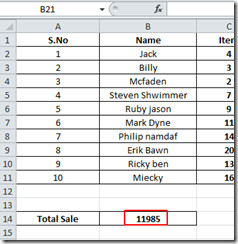Now we will simply convert the cell value to currency value, so we will move to Home tab, and From Number group, click the drop-down button, click Currency, as shown in the screenshot below.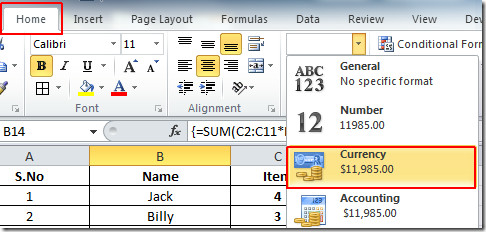This will the change the value in to default currency, as shown in the screenshot below.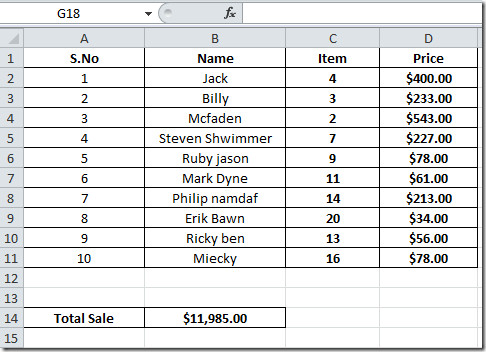You can also check out previously reviewed guides on Using REPT formula to create histogramUsing Payment Function In Excel.

1.johns says: